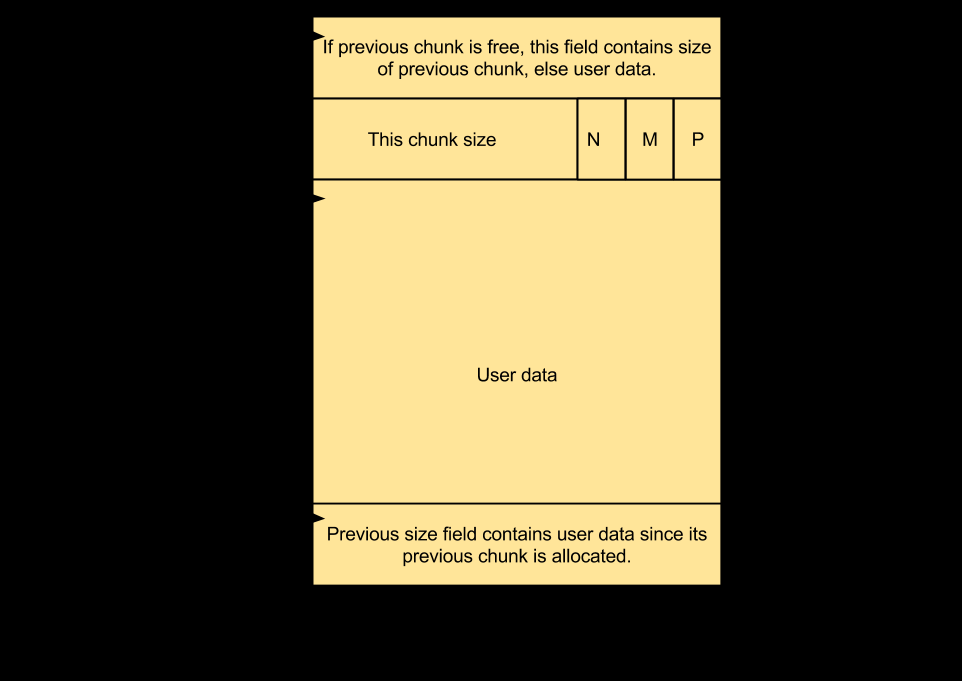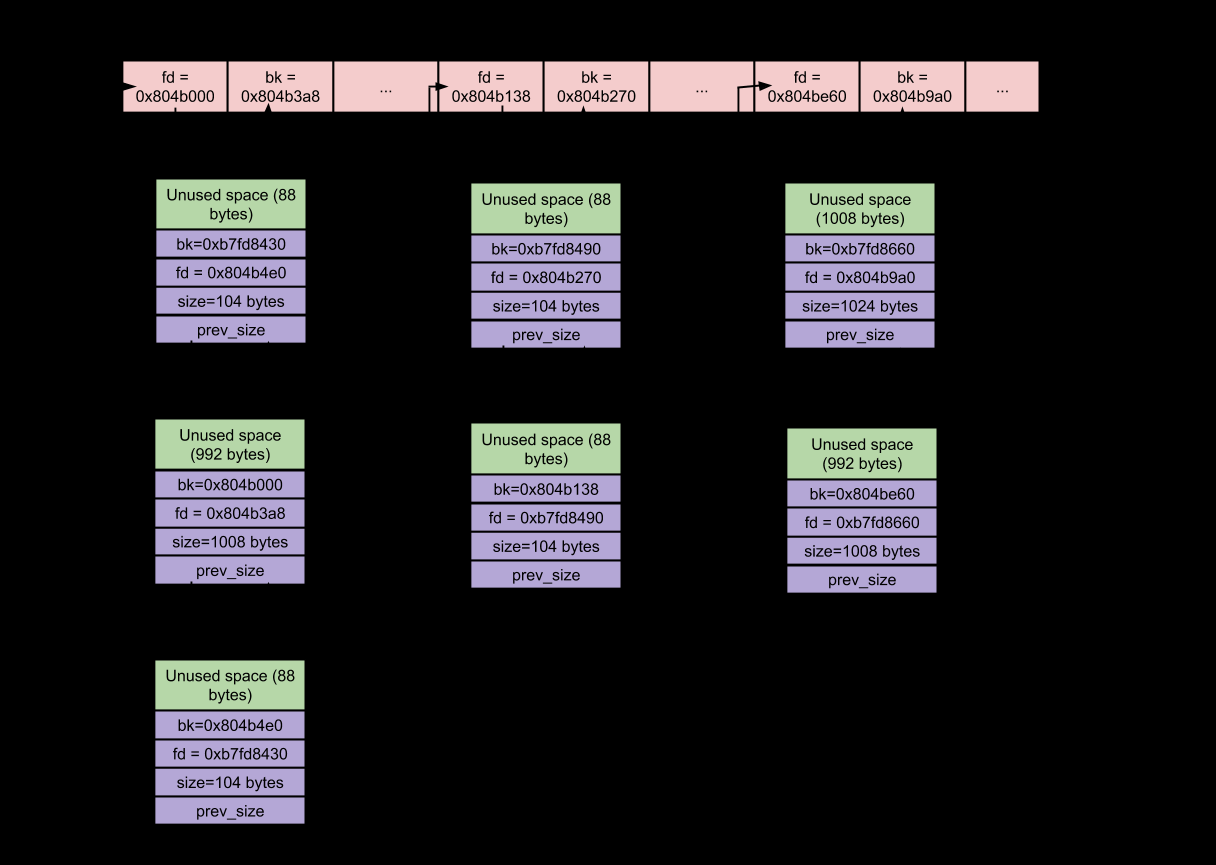# 0x00 前言

• 堆的内存怎样从内核中申请的？
• 怎样有效地进行内存管理？
• 堆内存是通过内核，库还是堆本身进行管理？
• 堆的一些相关问题能被利用吗？

• dlmalloc - 通用分配器
• ptmallac2- glibc
• jemalloc - FreeBSD & Firefox
• libumem - Solaris ...

# 0x02 示例：

``````#!c
/* Per thread arena example. */
#include <stdio.h>
#include <stdlib.h>
#include <unistd.h>
#include <sys/types.h>

getchar();
printf("After malloc and before free in thread 1\n");
getchar();
getchar();
}

int main() {
void* s;
int ret;

printf("Welcome to per thread arena example::%d\n",getpid());
getchar();
printf("After malloc and before free in main thread\n");
getchar();
getchar();
if(ret)
{
return -1;
}
if(ret)
{
return -1;
}
return 0;
}
``````

``````#!bash
Welcome to per thread arena example::6501
...
[email protected]/* <![CDATA[ */!function(t,e,r,n,c,a,p){try{t=document.currentScript||function(){for(t=document.getElementsByTagName('script'),e=t.length;e--;)if(t[e].getAttribute('data-cfhash'))return t[e]}();if(t&&(c=t.previousSibling)){p=t.parentNode;if(a=c.getAttribute('data-cfemail')){for(e='',r='0x'+a.substr(0,2)|0,n=2;a.length-n;n+=2)e+='%'+('0'+('0x'+a.substr(n,2)^r).toString(16)).slice(-2);p.replaceChild(document.createTextNode(decodeURIComponent(e)),c)}p.removeChild(t)}}catch(u){}}()/* ]]> */:~/ptmalloc.ppt/mthread\$ cat /proc/6501/maps
b7e05000-b7e07000 rw-p 00000000 00:00 0
...
[email protected]/* <![CDATA[ */!function(t,e,r,n,c,a,p){try{t=document.currentScript||function(){for(t=document.getElementsByTagName('script'),e=t.length;e--;)if(t[e].getAttribute('data-cfhash'))return t[e]}();if(t&&(c=t.previousSibling)){p=t.parentNode;if(a=c.getAttribute('data-cfemail')){for(e='',r='0x'+a.substr(0,2)|0,n=2;a.length-n;n+=2)e+='%'+('0'+('0x'+a.substr(n,2)^r).toString(16)).slice(-2);p.replaceChild(document.createTextNode(decodeURIComponent(e)),c)}p.removeChild(t)}}catch(u){}}()/* ]]> */:~/ptmalloc.ppt/mthread\$
``````

``````#!bash
Welcome to per thread arena example::6501
After malloc and before free in main thread
...
[email protected]/* <![CDATA[ */!function(t,e,r,n,c,a,p){try{t=document.currentScript||function(){for(t=document.getElementsByTagName('script'),e=t.length;e--;)if(t[e].getAttribute('data-cfhash'))return t[e]}();if(t&&(c=t.previousSibling)){p=t.parentNode;if(a=c.getAttribute('data-cfemail')){for(e='',r='0x'+a.substr(0,2)|0,n=2;a.length-n;n+=2)e+='%'+('0'+('0x'+a.substr(n,2)^r).toString(16)).slice(-2);p.replaceChild(document.createTextNode(decodeURIComponent(e)),c)}p.removeChild(t)}}catch(u){}}()/* ]]> */:~/lsploits/hof/ptmalloc.ppt/mthread\$ cat /proc/6501/maps
0804b000-0806c000 rw-p 00000000 00:00 0          [heap]
b7e05000-b7e07000 rw-p 00000000 00:00 0
...
[email protected]/* <![CDATA[ */!function(t,e,r,n,c,a,p){try{t=document.currentScript||function(){for(t=document.getElementsByTagName('script'),e=t.length;e--;)if(t[e].getAttribute('data-cfhash'))return t[e]}();if(t&&(c=t.previousSibling)){p=t.parentNode;if(a=c.getAttribute('data-cfemail')){for(e='',r='0x'+a.substr(0,2)|0,n=2;a.length-n;n+=2)e+='%'+('0'+('0x'+a.substr(n,2)^r).toString(16)).slice(-2);p.replaceChild(document.createTextNode(decodeURIComponent(e)),c)}p.removeChild(t)}}catch(u){}}()/* ]]> */:~/ptmalloc.ppt/mthread\$
``````

``````#!bash
Welcome to per thread arena example::6501
After malloc and before free in main thread
...
[email protected]/* <![CDATA[ */!function(t,e,r,n,c,a,p){try{t=document.currentScript||function(){for(t=document.getElementsByTagName('script'),e=t.length;e--;)if(t[e].getAttribute('data-cfhash'))return t[e]}();if(t&&(c=t.previousSibling)){p=t.parentNode;if(a=c.getAttribute('data-cfemail')){for(e='',r='0x'+a.substr(0,2)|0,n=2;a.length-n;n+=2)e+='%'+('0'+('0x'+a.substr(n,2)^r).toString(16)).slice(-2);p.replaceChild(document.createTextNode(decodeURIComponent(e)),c)}p.removeChild(t)}}catch(u){}}()/* ]]> */:~/lsploits/hof/ptmalloc.ppt/mthread\$ cat /proc/6501/maps
0804b000-0806c000 rw-p 00000000 00:00 0          [heap]
b7e05000-b7e07000 rw-p 00000000 00:00 0
...
[email protected]/* <![CDATA[ */!function(t,e,r,n,c,a,p){try{t=document.currentScript||function(){for(t=document.getElementsByTagName('script'),e=t.length;e--;)if(t[e].getAttribute('data-cfhash'))return t[e]}();if(t&&(c=t.previousSibling)){p=t.parentNode;if(a=c.getAttribute('data-cfemail')){for(e='',r='0x'+a.substr(0,2)|0,n=2;a.length-n;n+=2)e+='%'+('0'+('0x'+a.substr(n,2)^r).toString(16)).slice(-2);p.replaceChild(document.createTextNode(decodeURIComponent(e)),c)}p.removeChild(t)}}catch(u){}}()/* ]]> */:~/ptmalloc.ppt/mthread\$
``````

``````#!bash
Welcome to per thread arena example::6501
After malloc and before free in main thread
...
[email protected]/* <![CDATA[ */!function(t,e,r,n,c,a,p){try{t=document.currentScript||function(){for(t=document.getElementsByTagName('script'),e=t.length;e--;)if(t[e].getAttribute('data-cfhash'))return t[e]}();if(t&&(c=t.previousSibling)){p=t.parentNode;if(a=c.getAttribute('data-cfemail')){for(e='',r='0x'+a.substr(0,2)|0,n=2;a.length-n;n+=2)e+='%'+('0'+('0x'+a.substr(n,2)^r).toString(16)).slice(-2);p.replaceChild(document.createTextNode(decodeURIComponent(e)),c)}p.removeChild(t)}}catch(u){}}()/* ]]> */:~/ptmalloc.ppt/mthread\$ cat /proc/6501/maps
0804b000-0806c000 rw-p 00000000 00:00 0          [heap]
b7604000-b7605000 ---p 00000000 00:00 0
b7605000-b7e07000 rw-p 00000000 00:00 0          [stack:6594]
...
[email protected]/* <![CDATA[ */!function(t,e,r,n,c,a,p){try{t=document.currentScript||function(){for(t=document.getElementsByTagName('script'),e=t.length;e--;)if(t[e].getAttribute('data-cfhash'))return t[e]}();if(t&&(c=t.previousSibling)){p=t.parentNode;if(a=c.getAttribute('data-cfemail')){for(e='',r='0x'+a.substr(0,2)|0,n=2;a.length-n;n+=2)e+='%'+('0'+('0x'+a.substr(n,2)^r).toString(16)).slice(-2);p.replaceChild(document.createTextNode(decodeURIComponent(e)),c)}p.removeChild(t)}}catch(u){}}()/* ]]> */:~/ptmalloc.ppt/mthread\$
``````

``````#!bash
Welcome to per thread arena example::6501
After malloc and before free in main thread
After malloc and before free in thread 1
...
[email protected]/* <![CDATA[ */!function(t,e,r,n,c,a,p){try{t=document.currentScript||function(){for(t=document.getElementsByTagName('script'),e=t.length;e--;)if(t[e].getAttribute('data-cfhash'))return t[e]}();if(t&&(c=t.previousSibling)){p=t.parentNode;if(a=c.getAttribute('data-cfemail')){for(e='',r='0x'+a.substr(0,2)|0,n=2;a.length-n;n+=2)e+='%'+('0'+('0x'+a.substr(n,2)^r).toString(16)).slice(-2);p.replaceChild(document.createTextNode(decodeURIComponent(e)),c)}p.removeChild(t)}}catch(u){}}()/* ]]> */:~/ptmalloc.ppt/mthread\$ cat /proc/6501/maps
0804b000-0806c000 rw-p 00000000 00:00 0          [heap]
b7500000-b7521000 rw-p 00000000 00:00 0
b7521000-b7600000 ---p 00000000 00:00 0
b7604000-b7605000 ---p 00000000 00:00 0
b7605000-b7e07000 rw-p 00000000 00:00 0          [stack:6594]
...
[email protected]/* <![CDATA[ */!function(t,e,r,n,c,a,p){try{t=document.currentScript||function(){for(t=document.getElementsByTagName('script'),e=t.length;e--;)if(t[e].getAttribute('data-cfhash'))return t[e]}();if(t&&(c=t.previousSibling)){p=t.parentNode;if(a=c.getAttribute('data-cfemail')){for(e='',r='0x'+a.substr(0,2)|0,n=2;a.length-n;n+=2)e+='%'+('0'+('0x'+a.substr(n,2)^r).toString(16)).slice(-2);p.replaceChild(document.createTextNode(decodeURIComponent(e)),c)}p.removeChild(t)}}catch(u){}}()/* ]]> */:~/ptmalloc.ppt/mthread\$
``````

``````#!bash
Welcome to per thread arena example::6501
After malloc and before free in main thread
After malloc and before free in thread 1
...
[email protected]/* <![CDATA[ */!function(t,e,r,n,c,a,p){try{t=document.currentScript||function(){for(t=document.getElementsByTagName('script'),e=t.length;e--;)if(t[e].getAttribute('data-cfhash'))return t[e]}();if(t&&(c=t.previousSibling)){p=t.parentNode;if(a=c.getAttribute('data-cfemail')){for(e='',r='0x'+a.substr(0,2)|0,n=2;a.length-n;n+=2)e+='%'+('0'+('0x'+a.substr(n,2)^r).toString(16)).slice(-2);p.replaceChild(document.createTextNode(decodeURIComponent(e)),c)}p.removeChild(t)}}catch(u){}}()/* ]]> */:~/ptmalloc.ppt/mthread\$ cat /proc/6501/maps
0804b000-0806c000 rw-p 00000000 00:00 0          [heap]
b7500000-b7521000 rw-p 00000000 00:00 0
b7521000-b7600000 ---p 00000000 00:00 0
b7604000-b7605000 ---p 00000000 00:00 0
b7605000-b7e07000 rw-p 00000000 00:00 0          [stack:6594]
...
[email protected]/* <![CDATA[ */!function(t,e,r,n,c,a,p){try{t=document.currentScript||function(){for(t=document.getElementsByTagName('script'),e=t.length;e--;)if(t[e].getAttribute('data-cfhash'))return t[e]}();if(t&&(c=t.previousSibling)){p=t.parentNode;if(a=c.getAttribute('data-cfemail')){for(e='',r='0x'+a.substr(0,2)|0,n=2;a.length-n;n+=2)e+='%'+('0'+('0x'+a.substr(n,2)^r).toString(16)).slice(-2);p.replaceChild(document.createTextNode(decodeURIComponent(e)),c)}p.removeChild(t)}}catch(u){}}()/* ]]> */:~/ptmalloc.ppt/mthread\$
``````

# 0x03 Arena

Arena的数量：在以上示例中，我们看到了主线程包含 main arena 同时 thread 1 包含了它自己的 thread arena。所以不论线程数量，在线程与 arena 之间可以有一对一的映射吗？当然没有。一个 arena 应用程序可以包含更多数量的线程（相较于 Cores 的数量），在这样的情况下，每个线程拥有一个 arena 显得有些不值。因此，应用程序的arena 数量的限制是基于系统里现有的 Cores 的数量

``````#!bash
For 32 bit systems:
Number of arena = 2 * number of cores.
For 64 bit systems:
Number of arena = 8 * number of cores.
``````

# 0x05 Multiple Heaps：

heap_info – 堆头 – 单个 thread arena 有多个堆。每个堆有它自己的头。为什么需要多个堆？每个 thread arena 开始都只有一个堆，但当这个堆耗尽空间时，新的堆（并不连续的）能 mmap 到这个 arena 里。

malloc_state – Arena 头 – 单个 thread arena 有多个堆，但是对于所有这些堆来说，只存在一个 arena 头。arena 头 包含 bins、top chunk、最后剩余块……

malloc_chunk – 块头 – 一个堆基于用户请求被分为很多块。每个块有它自己的块头。

Main arena 没有多重堆，因此没有 heap_info 结构。当main arena 耗尽空间时，sbrk 堆被扩展（连续区域）直到它转储到内存映射分段中。

main arena 和 thread arena 的形象化视图（单个堆分段）：Chunk ：在堆里的块可以是以下几种类型中的一种：

• Allocated chunk 分配后的块
• Free chunk 空闲块
• Top chunk 开始块
• Last Remainder chunk 最后剩余块

# 0x06 Allocated Chunk：size: 这部分包含了此处 Allocated 块的容量大小，该部分最后 3 bits 包含了关键信息。 PREV_INUSE (P) – 这一 bit 在前一个块被分配时就被设置。 IS_MMAPPED (M) – 这一 bit 在块即在 mmap 时被设置。 NON_MAIN_ARENA (N) – 这一 bit 在块分配给 thread arena 时设置。

Free Chunk（空闲块）:

prev_size： 两个空闲块是不能毗连在一起的。当两个块都空闲时，它就会与一个单独的空闲块连接。因此前一个块及当前这个释放的块会被分配，同时 prev_size 包含前一个块的用户数据。 size: 这个部分包含有空闲块的 size。 fd: 前指针 – 同一容器里指向下一块的指针（不是指向物理内存内的下一块）。 Bins：Bins 是 freelist 数据结构。用以支持 Free Chunk。基于块的大小，有几种可用的 bins：

Fast bin 快速bin Unsorted bin 无序bin Small bin 小bin Large bin 大bin

fastbinsY: 该数组支持 fast bins bins: 该数组支持 unsorted，small 以及 large 容器。总共 126 个容器被划分为以下几种： Bin 1 – Unsorted bin Bin 2 to Bin 63 – Small bin Bin 64 to Bin 126 – Large bin

Fast Bin: 大小在 1680 bytes 的块叫做 fast chunk 。支持 fast chunk 的 bin 叫做 fast bins。在上述的这些 bin 里，fast bins 在内存分配和重新分配上更快。（译者注：只有 fast bin 是 LIFO 其他的 bin 都是 FIFO）

bin 数量 - 10

Chunk size – 8 bytes apart 块大小（size） - 8 bytes 累加

Fast bins 包含大小为 8 bytes 累加的块的 binlist 。即，第一个 fast bin(index 0) 包含 16 bytes 块的binlist，第二个 fast bin (index 1)包含 24 bytes 以此类推……（译者注：binlist 是 bin 的一个链表） 在一个独有的 fast bin 内的块大小是一样的。

malloc 初始化 过程中，最大的 fast bin 的大小设置64 (!80) bytes。因此通过块的默认大小设置为 16 到 64 就可以将其划分为 fast chunks 了。 不能合并 - 空闲的两个块可以相邻，但不能合并为一个空闲块。不能合并导致产生外存储碎片，但它可以大大提速！！ malloc(fast chunk) –

free(fast chunk) –

Unsorted Bin: 当小或者大的块被释放而非把他们添加到各自 bin 里时，它会被添加到 unsorted bin 里。 该途径给了 "glibc malloc" 第二个机会再次利用最近释放的块。因此内存分配以及回收的速度都会有所提升（因为 unsorted bin）由于排除了用于查找合适容器的时间。

Number of bins – 1

Unsorted bin 包含空闲块的一个循环双向链表（也称为 binlist 容器列表）。 块的大小 - 没有大小限制，任何大小的块都属于此容器。Small Bin: 小于 512 bytes 的块叫做 small chunk。 支持 small chunks 的容器叫做 small bins。 Small bins 在内存分配和回收时比 large bins 快（比 fast bins 慢）。

Number of bins – 62

• 每个 small bin 含有空闲块的一个循环双向链表（也称为 binlist 容器列表）。在 small bins 里， 块从列表中间断开链接后，便使用双向链表。在这里列表头部实现添加并在列表的后部删除 - FIFO（先进先出）。

Chunk Size – 8 bytes 递增

• Small bin 包含一个块大小为 8 bytes 递增（即，第一个 Small Bin（Bin 2）包含块大小为 16 bytes 的binlist，第一个 Small Bin (Bin 3)包含块大小为 24 bytes 的 binlist 以此类推）的 binlist。
• 一个 small bin 里的块大小是一样的，因此不需要分类。

malloc(small chunk) – * 初始状态下所有 small bins 都是 NULL，因此即使用户请求一个 small chunk，提供服务的是 unsorted bin code 而不是 small bin code。 * 在第一次调用 malloc 期间，在 malloc_state 里发现的 small bin 和 large bin 数据结构（bins 容器）被初始化（即，bins 会指向它自己表示他们是空的）。 * 之后当 small bin 处于非空状态时，其对应 binlist 中的最后一个块会被移除返回给用户。 free(small chunk) – * 释放块时，查看其前一个或下一个块是否空闲，如果空闲就合并（即，从他们各自的链表里解除块的链接，然后在 unsorted bin 的链表最开端添加新的统一的块）。

Large Bin: 大小大于等于 512 bytes 的块称为 large chunk。支持 large chunks 的 bins 叫作 large bins。Large bins 在内存分配和回收中比 small bins 要慢。

Number of bins – 63 每个 large bin 包含空闲块的一个循环双向链表（也称为 binlist ）。在 large bins 里，块在任何位置（前端或中间或尾部）被添加或移除后，开始使用双向链表。 这 63 bins 以外：

• 32 bins 包含大小为 64 bytes 累加的块的 binlist。即，第一个 large bin（Bin 65）包含大小为 512 bytes 到 568 bytes 的块的 binlist，第二个 large bin（Bin 66）包含大小为 576 到 632 bytes 的块的 binlist 以此类推……
• 16 bins 包含着大小为 512 bytes 累加的块的 binlist。
• 8 bins 包含着大小为 4096 bytes 累加的块的 binlist。
• 4 bins 包含大小为 32768 bytes 累加的块的 binlist。
• 2 bins 包含大小为 262144 bytes 累加的块的 binlist。
• 1 bin 包含一个拥有剩余容量大小的块。

malloc(large chunk) –

free(large chunk) – 它的程序与空闲（small chunk）类似。

Top Chunk: 在一个 arena 顶部边框的块叫做开始块(Top Chunk)。它不属于任何容器。在任一 bin 里，Top Chunk被用于在没有空闲块时为用户请求提供服务。